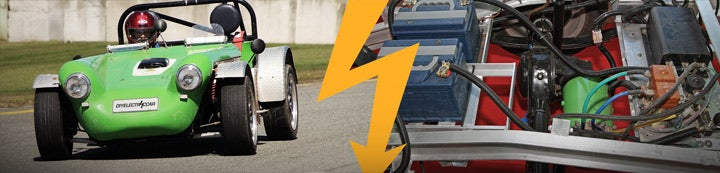1 - 1 of 1 Posts

#### EVDL List

·
##### Registered
Joined
·
70 Posts
Discussion Starter · ·
Re: [EVDL] Magnetic Shock Absorber & EV

David Roden <[email protected]> wrote:
> On 6 Oct 2007 at 22:59, Morgan LaMoore wrote:
>
> > Energy in 16 T-105's (using 6 hour rate even though he's at 3 hour
> > rate, ignoring Peukert's effect):
> > 16*6V*205Ah
> > 19.7 kWh
>
> He's using T-145 batteries. But let's be realistic here and use the 3hr
> rating of 188ah for them (from Uve's calculator). Now we have only 18kWh.

Yeah, I stated that I was making some assumptions that really enlarge
the capacity. Your number is probably much more realistic.

> >.5*.35*30ft^2*1.3kg/m^3*(55mph)^2*150miles
> > 25.7 kWh
>
> I have the wrong degree for this, so I may be blowing smoke - but aren't you
> mixing your units here? You have kg/m^3 but you use miles for the distance.
> I can't seem to get the same figures you did; my numbers are wildly
> different.
>

I don't have an Aero degree either, but I studied drag in physics
recently. The equation is F=1/2*Cd*A*rho*v^2, and energy is force
times distance (for a constant force). I just put everything into the
equation in the units I had. I used Google Calculator and let it do
the conversions for me. I used this string:

.5*.35*30ft^2*1.3kg/m^3*(55mph)^2*150miles in kW hours

and it returns the result. My calculator can also do this, but it's
easier to type it into Google.

If I wanted to, I could have converted everything to SI first:

30 ft^2 * (1609 m/5280 ft)^2=2.79m^2
55mph * (1609m/mile)/(3600s/hour)=24.6m/s
150miles * (1609m/mile) = 241350m
.5*.35*2.79m^2*1.3kg/m^3*(24.6m/s)^2*(241350m)=92705000J=92.7MJ
92.7MJ/(3.6MJ/kWh) = 25.75kWh

It's just easier to let a computer handle units for you. Plus, my
memorized conversion factors and intermediate rounding introduced an
error of 0.2% (not that that really matters; my original figures
aren't that accurate anyways).

-Morgan LaMoore

_______________________________________________
For subscription options, see
http://lists.sjsu.edu/mailman/listinfo/ev

1 - 1 of 1 Posts